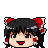# POJ 2823. Sliding Window

### Brief description:

。。维护一个宽度为 k 的滑动窗口。。分别统计所有位置的最大值和最小值。。

# SRM 491

500 :

1：数字范围在[1，N]之间且两两不相同。
2：对面数字和相等且 >= k。
3：经过旋转相同的筛子被看作是等价的。

（room 里有人用DP?.. 是怎么DP的呢？）

1000 :

#include
using namespace std;

int Cn3(int n){
if (n < 3) return 0;
return n * (n-1) * (n-2) / 6;
}

class FoxMakingDiceEasy{
public:
int theCount(int n, int k){
int res = 0, MAX = n * 2 - 5;
// i: 为了计算，枚举正反两面的数字和 - 1 的值。
for (int i=k-1;i
#include
#include
using namespace std ;

class BottlesOnShelf
{
public :
long long  gcd( long long a , long long b ) { if ( b == 0 ) return a ; return gcd(b , a%b) ; }
long long  lcm( long long a , long long b ) { long long c = gcd(a , b) ; return a * b / c ; }

int getNumBroken(int N, vector  L, vector  R, vector  D)
{
int M = L.size() , p , ll , rr , ans ;
long long w , c ;

ans = 0 ;
for ( int i = 1 ; i < (1 << M) ; i ++ )
{
p = 0 ;
for ( int j = 0 ; j < M ; j ++ ) if ( ( i >> j ) & 1 ) p ++ ;

ll = 1 ; rr = N ; w = -1 ;
for ( int j = 0 ; j < M ; j ++ ) if ( ( i >> j ) & 1 )
{
if ( L[j] > ll ) ll = L[j] ;
if ( R[j] < rr ) rr = R[j] ;
if ( w == -1 ) w = D[j] ; else w = lcm(w , (long long)D[j]) ;
}

c = (long long)(rr / w) - (long long)((ll-1) / w) ;
if ( p % 2 == 1 ) ans += c ; else ans -= c ;
}
return ans ;
}
} ;



# Nim Game

### 序

Nim Game问题是博弈问题中的重头戏，也是现在信息学竞赛中考察最多的。Nim Game往往与xor运算相联系，同时又引出了很多的分支，最经典的是Nim-k、anti-Nim、Staircase Nim、take&break四个拓展问题。此外还有Misère Nim，概率问题。ゆっくり読んでください …